### Home > CALC > Chapter 3 > Lesson 3.2.3 > Problem3-80

3-80.
1. The graph below shows the height of a soccer ball after being kicked straight up into the air. Homework Help ✎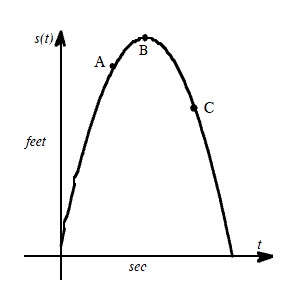1. Explain why the slope of the tangent at point A will determine the velocity of the ball at that point.

2. At which of the labeled points is the velocity the greatest? How can you tell?

3. At what point does the ball momentarily stop? What is the velocity at this point?

4. The distance from the ground can be described by the function s(t) = −l6t2 + 76.8t + 5. Find v(t) = s'(t).

5. Find the instantaneous velocity at t = 2, 3, and 4 seconds.

6. What does negative velocity represent in this problem?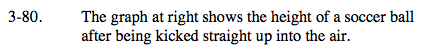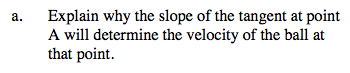Since the slope of a secant determines the Average Velocity (between two points),the slope of a tangent will determine the Instantaneous Velocity (at one point).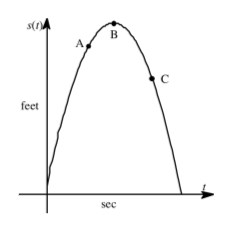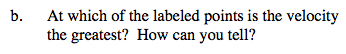Be sure to consider the difference between velocity and speed.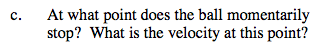Since velocity changes from positive to negative continuously, and since 0 is in between positive and negative values, the Intermediate Value Theorem guarantees that velocity will (at one moment) be 0; therefore, the ball will momentarily stop.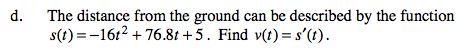Use the Power rule.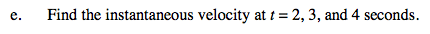Use your derivative function in part (d) to find v(2), v(3) and v(4).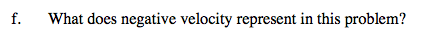What is happening to the soccer ball when the velocity is negative?